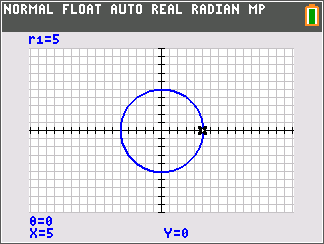••• ##### Device
• TI-84 Plus
• TI-84 Plus Silver Edition
•TI-84 Plus C Silver Edition
•TI-84 Plus CE

# Precalculus: Transitions

by Texas Instruments#### Overview

In this activity, students review some basic relationships relating to the unit circle and apply these relationships in the conversion of a rectangular circle equation to polar form.

#### Key Steps

•Students convert rectangular equations to polar form and vice versa. Prior knowledge of the unit circle is applied in obtaining key equations useful in making the conversions.

•Students will test their results by graphing both the rectangular and polar forms. If the results are equivalent, as long as the graphing window is set at the same values, the graphs will appear the same.